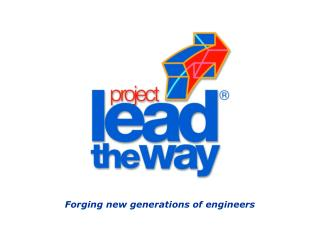DownloadDownload PresentationForging new generations of engineers

# Forging new generations of engineers

Download Presentation## Forging new generations of engineers

- - - - - - - - - - - - - - - - - - - - - - - - - - - E N D - - - - - - - - - - - - - - - - - - - - - - - - - - -
##### Presentation Transcript

1. Forging new generations of engineers

2. Math for Truss Calculation

3. 500 N B 500 N 2m 45 A C AX 2m AY CY Trusses Trusses are structures made up of beams joined together at their endpoints. Solve for the force in each member of the truss in this example to find whether the members are in tension or compression. Start be replacing the supports with reaction forces:

4. 500 N 500 N AX AX AY CY AY CY Trusses • This truss consists of: • 2 unknown member forces and 1 unknown external force at joint B • 2 unknown member forces and 2 unknown reaction forces at joint A • 2 unknown member forces and 1 unknown reaction force at joint C • Draw the free-body diagrams for each pin:

5. 500 N 500 N FBC FAB FBC FAB AX FAC FAC AX AY CY CY AY Trusses • These three separate free-body diagrams assume that: • Member AB is in tension • Member BC is in compression • Member AC is in tension • If these assumptions are incorrect, then our solution will show a negative quantity for force.

6. Trusses • To really “see” that • Member AB is in tension • Member BC is in compression • Member AC is in tension • We must look at the free-body diagrams of the beams, which show the effects of the pins on the beams.

7. FBC FBCY 45 FBCX Trusses Before we can solve for the forces, we must break FBC into its x and y components: FBCX = FBC * cos (45) FBCY = FBC * sin (45)

8. FBC sin(45) FBC cos(45) 500 N FAB Trusses + FX = 0 ; 500N – FBCcos(45) = 0 FBCcos(45) = 500N cos(45) cos(45) FBC = 707.1 N (C) +FY = 0 ; FBCsin(45) – FAB = 0 707.1*sin(45) – FAB = 0 FAB = 707.1*sin(45) FAB = 500 N (T) The following is the free-body diagram of joint B. The force FBC has been replaced with its x and y components:

9. Trusses + FX = 0 ; 500N – FBCcos(45) = 0 FBCcos(45) = 500N cos(45) cos(45) FBC = 707.1 N (C) +FY = 0 ; FBCsin(45) – FAB = 0 707.1*sin(45) – FAB = 0 FAB = 707.1*sin(45) FAB = 500 N (T) The (T) indicates tension and the (C) indicates compression. Both solutions were positive therefore our initially assumptions were correct. (If the solution had been a negative number, then we would simply reverse our assumption from tension to compression or vice versa)

10. FBC 45 FAC CY Trusses + FX = 0 ; – FAC+ FBC cos (45) = 0 – FAC+ 707.1 cos (45) = 0 FAC = 707.1 cos(45) FAC = 500 N (T) +FY = 0 ; CY – FBC *sin(45)= 0 CY – 707.1*sin(45)= 0 CY = 500 N The following is the free-body diagram of joint C. The force FBC has been replaced with its x and y components:

11. FAB AX FAC AY Trusses + FX = 0 ; FAC - Ax = 0 500 N - Ax = 0 AX = 500 N +FY = 0 ; FAB - AY = 0 500 N - AY = 0 AY = 500 N The following is the free-body diagram of joint A:

12. 500 N 500 N B 2m 500 N (T) 707.1 N (C) 45 500 N A C 500 N (T) 2m 500 N 500 N Trusses Solved!!!